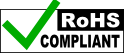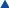## RPM sensor, hall effect, includes magnets

### \$30.00 USD

Part: APRS6569
Weight: 0.25 lbsHall effect RPM sensor. Includes two small ceramic discs magnets. Registers pulse counts off of a magnet to measure rotational speed for wind turbine testing. Short pigtail leads attached. Can be configured with longer leads for additional cost.

## Datasheet

Technical data sheet from the manufacturer of the sensor.

## Usage with Wind Data Logger:

The RPM sensor can be connected to one of the anemometer channels as you would with a normal anemometer.

Your calibration values values for calculating RPM and total daily revolutions would be as follows (n = number of magnets used).

```RPM:
Anemometer m (multiplier) = 60 / n
Anemometer b (offset) = 0

Total Daily Revolutions:
Counter m (multiplier) = 1 / n
Counter b (offset) = 0
```

So for an example, if you used Anemometer Channel 2 and two magnets, you could do the following:

```Main Setup Menu > Anemometer Setup > Anemometer 2

AN_M2 = +0030.0000
AN_B2 = +0000.0000
Label2 = TURBINE RPM
AN2_UNIT = RPM

Main Setup Menu > Counter Setup > Counter 2

CNT_M2 = +0000.5000
CNT_B2 = +0000.0000
Label2 = TOTAL REVS
CNT2_UNIT = CNT
```

back to top